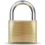# Template:Col-end

|}This high-risk template has been protected from editing to prevent vandalism. Please discuss changes on the talk page or request unprotection. You may use the talk page to ask an administrator to make an edit for you.

This Template is a self-reference and so is part of the Wikipedia project rather than the encyclopaedic content.

## Usage

A border of 2px (1px width on each side) corresponds to a 5%. Therefore, with a 2px border — like on Wikipedia:Tutorial (Wrap-up and more info) — the width needs to be 95% for the table to fit within the screen. Since it's possible that some tables might not have a border, correction on the individual page could be made after substitution. Another way do a two column table is like this:

 ```{{col-begin|width=95%}} |- | Left column | Right column |} ```

 ```{{Col-begin}} {{Col-1-of-2}} Column 1 here {{Col-2-of-2}} Column 2 here {{Col-end}} ``` ```{{Col-begin}} {{Col-1-of-3}} Column 1 here {{Col-2-of-3}} Column 2 here {{Col-3-of-3}} Column 3 here {{Col-end}} ``` ```{{Col-begin}} {{Col-1-of-4}} Column 1 here {{Col-2-of-4}} Column 2 here {{Col-3-of-4}} Column 3 here {{Col-4-of-4}} Column 4 here {{Col-end}} ```

 ```{{Col-begin|width=}} {{Col-1-of-5}} Column 1 here {{Col-2-of-5}} Column 2 here {{Col-3-of-5}} Column 3 here {{Col-4-of-5}} Column 4 here {{Col-5-of-5}} Column 5 here {{Col-end}} ``` ```{{Col-begin|bgColor=transparent}} {{Col-1-of-6}} Column 1 here {{Col-2-of-6}} Column 2 here {{Col-3-of-6}} Column 3 here {{Col-4-of-6}} Column 4 here {{Col-5-of-6}} Column 5 here {{Col-6-of-6}} Column 6 here {{Col-end}} ``` ```{{Col-begin|class=references-small}} {{Col-break}} Column 1 here {{Col-break}} Column 2 here {{Col-break}} Column 3 here {{Col-break}} Column 4 here {{Col-break}} Column 5 here {{Col-break}} Column 6 here {{Col-end}} ```

 ```{{Top}} Column 1 here {{Mid}} Column 2 here {{Bottom}} ``` ```{{Columns-start|num=3}} Column 1 here {{Column}} Column 2 here {{Column}} Column 3 here {{Columns-end}} ``` ```{{Multicol}} Column 1 here {{Multicol-break}} Column 2 here {{Multicol-break}} Column 3 here {{Multicol-break}} Column 4 here {{Multicol-end}} ```